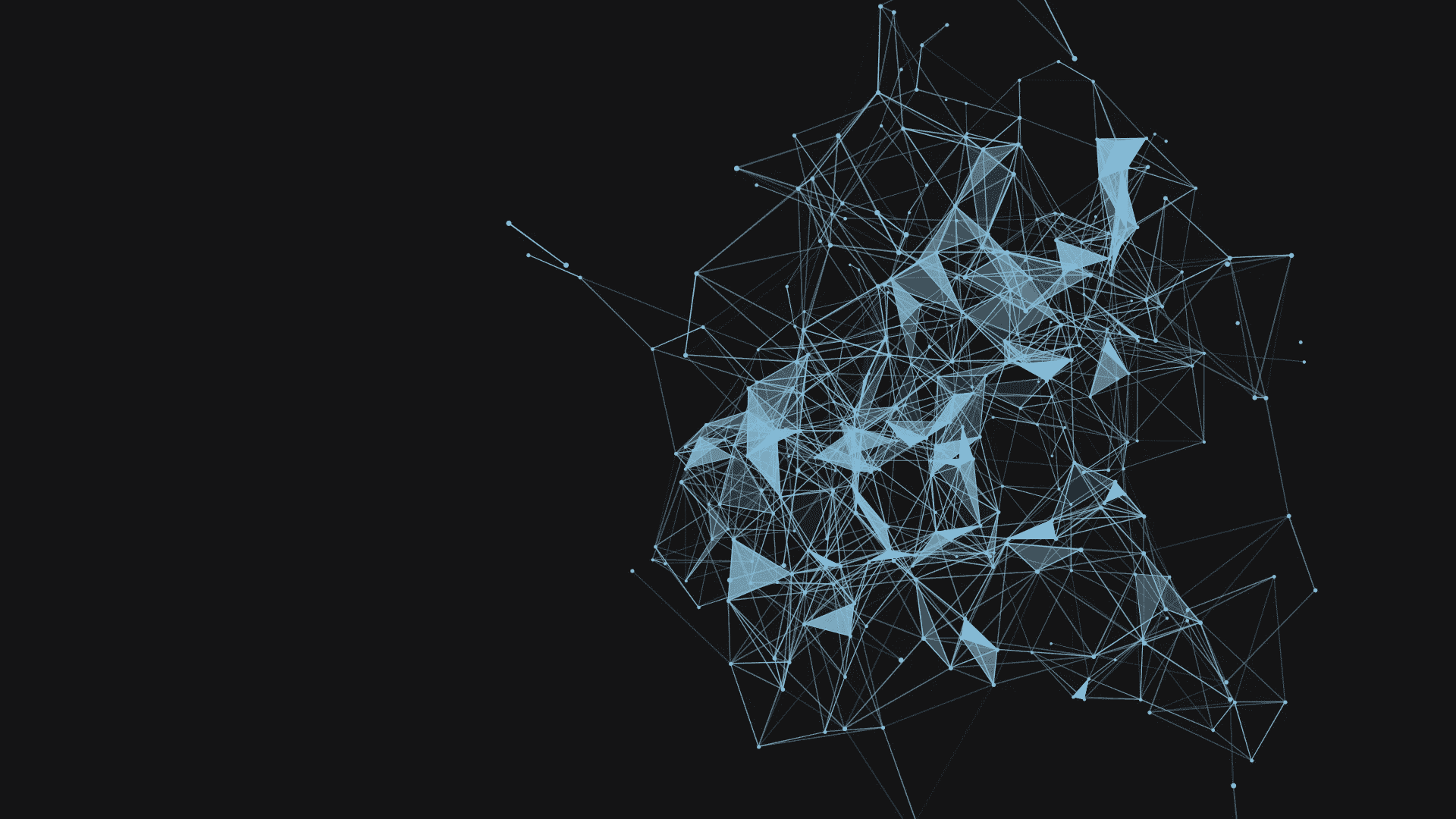# Debunking Artificial Neural Networks (ANN) with practical examples

Source: Deep Learning on MediumThis post is divided into 3 sections each with its own problem statement.

# 1. Regression:

Regression is about predicting a real OR continuous quantity such as ‘salary’ or ‘price’ or ‘weight’. Predicting the price of a stock at a given time based on the previous pattern, predicting the price of a real estate property into the future, predicting the salary of an employee based on data from a previous company, etc can be categorized into Regression problems. The Regression problem comes under the Supervised Learning category. In ML there are Linear models to solve Linear problems and Non-Linear models to solve Non-Linear problems. I would highly suggest you go through these models to get a better understanding of the Math behind them. It also helps in building up the intuition. Here we will try to solve a Simple Linear Regression problem with ANN.

The code in itself is quite well commented from my side but we will still try to simplify it by breaking it down piece by piece

## Breaking down the code:

Lines 1–8 deals with importing all the necessary modules and libraries. Numpy is used for numerical computations, Pandas for data manipulation, Sklearn for data creation and preprocessing and finally Keras for creating the ANN model.

`import numpy as npimport pandas as pdfrom keras.models import Sequentialfrom keras.layers import Dense, Dropoutfrom sklearn.preprocessing import MinMaxScalerfrom sklearn.datasets import make_regression`

Line 10–21 deals with creating a random blob of data suitable for making a regression prediction and scaling it. Scaling the input data is a necessary step in some datasets because of its high variability. Scaling the dataset makes the model less prone to outliers.

`X, Y = make_regression(n_samples=100, n_features=4, noise=0.1, random_state=1)scaled_X = MinMaxScaler()scaled_Y = MinMaxScaler()scaled_X.fit(X)scaled_Y.fit(Y.reshape(100, 1))X = scaled_X.transform(X)Y = scaled_Y.transform(Y.reshape(100, 1))`

On line 23–24 we initialize the instance of a model

`model = Sequential()`

From lines 26–42 we actually create our ANN model with Dense networks. Notice after every layer of a dense neural network we add another line with the Dropout method. The Dropout method in Keras randomly drops nodes from the layer. This is done in order to avoid overfitting in our model. Overfitting means that the model does not learn from the feature input but instead, it starts remembering the entire data destroying the model’s generalization capability. The last Dense layer will contain only one node which corresponds to only one real-valued output of the regression task.

`model.add(Dense(units = 32, input_dim = 4, activation = 'relu'))model.add(Dropout(0.10))model.add(Dense(units = 32, activation = 'relu'))model.add(Dropout(0.20))model.add(Dense(units = 32, activation = 'relu'))model.add(Dropout(0.20))model.add(Dense(units = 1, activation = 'linear'))`

In the next five lines, we compile the created model with a few parameters like losses, optimizers, etc. Let’s try to briefly understand what some of these terms mean.

1. Loss- Also known as cost function or error function is a method to evaluate the model’s prediction capability. The higher the loss function the lower the model’s performance. Available losses in Keras
2. Optimizer- Training a Neural network is all about minimizing the cost function and also minimizing the difference between actual output and expected output. Optimizers tie together the loss function and model parameters by updating the model in response to the output of the loss function. Available Optimizers in Keras
3. Epoch- Number of iteration for which the Neural Network is trained before finalizing the output.
`model.compile(loss='mse', optimizer='adam', metrics = ['mae'])model.fit(X, Y, epochs = 100, verbose = 0)`

I would still press on reading this article thoroughly for a better understanding of all of these important ML terminologies.

That’s it. Our model is ready to make predictions. In the last part of this code, we create yet another blob of random data, one that the model has not been trained on, and test our small yet powerful model on it to see how it performs.

`Xnew, a = make_regression(n_samples=3, n_features=4, noise=0.1, random_state=1)Xnew = scaled_X.transform(Xnew)ynew = model.predict(Xnew)for i in range(len(Xnew)): print("X =",Xnew[i], ",", "Predicted =",ynew[i])`